GFG App
Open AppBrowser
Continue

# How to Fix in R: object of type ‘closure’ is not subsettable

In this article, we will discuss how to fix the “object of type ‘closure’ is not subsettable” error in the R programming language.

The error that one may face in R is:

`object of type 'closure' is not subsettable`

The R compiler produces such an error when we try to subset a function. In R, we can subset a list, vector, etc but a function having a type ‘closure that can’t be subsetted.

## When this error might occur:

Consider that we have a function in R that takes each element of a vector as input and add it with 10:

## R

 `# Define a function` `Add <- ``function``(x) {` `  ``x <- x + 10` `  ``return``(x)` `}`

## R

 `# Define a function` `Add <- ``function``(vect) {` `  ``vect <- vect + 10` `  ``return``(vect)` `}`   `# Define a vector` `vect <- ``c``(8, 9, 12, 14, 20)`   `# Calling Add function` `Add``(vect)`

Output:As you can see in the output, each element of the vector is added with 10. Now, suppose we try to subset this function:

## R

 `# Define a function` `Add <- ``function``(vect) {` `  ``vect <- vect + 10` `  ``return``(vect)` `}`   `# Define a vector` `vect <- ``c``(8, 9, 12, 14, 20)`   `# Try to access the second element` `# of the function` `Add`

Output: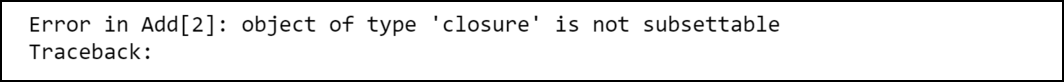The compiler produces such an error because it is not allowed to subset an object having the type closure in R. In order to confirm that the function is of type closure we can use the typeof() function that has the following syntax:

Syntax: typeof(x)

Parameters:

x: It represents the specified data

## R

 `# Define a function` `Add <- ``function``(vect) {` `  ``vect <- vect + 10` `  ``return``(vect)` `}`   `# Define a vector` `vect <- ``c``(8, 9, 12, 14, 20)`   `# Print the type` `typeof``(Add)`

Output: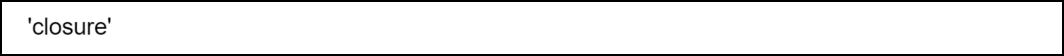Any function in R is of type closure. For example, let’s try to subset a median() inbuilt function first:

Syntax: median(x)

Parameter: x: a vector

Return Type: Returns the median of vector elements

## R

 `# Try to access the first element` `median`

Output: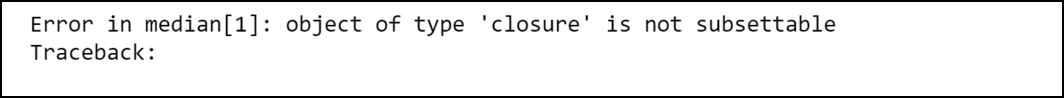Now we will subset the range() inbuilt function:

Syntax: range(x)

Parameter: x: a vector

Return Type: Returns a range

## R

 `# Try to access the first element` `range`

Output:## How to fix this error:

We can fix this error easily by not subsetting the function. For example, suppose we want to use the Add() function for the second element of the vector vect:

## R

 `# Define a function` `Add <- ``function``(vect) {` `  ``vect <- vect + 10` `  ``return``(vect)` `}`   `# Define a vector` `vect <- ``c``(8, 9, 12, 14, 20)`   `# Try to access the second element ` `# of the function` `Add``(vect)`

Output: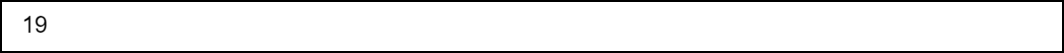This time the program compiled successfully because here we are subsetting the vector instead of the function. Also, note that we can apply the Add() on the entire vector:

## R

 `# Define a function` `Add <- ``function``(vect) {` `  ``vect <- vect + 10` `  ``return``(vect)` `}`   `# Define a vector` `vect <- ``c``(8, 9, 12, 14, 20)`   `# Calling Add function` `Add``(vect)`

Output: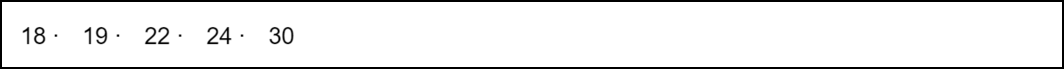My Personal Notes arrow_drop_up